Question

Given the thermodynamic data below, calculate the value of the equilibrium constant for the reaction shown...

Given the thermodynamic data below, calculate the value of the equilibrium constant for the reaction shown at 25.0ºC

H₂ (g) + I₂ (g) ⇄ 2 HI (g)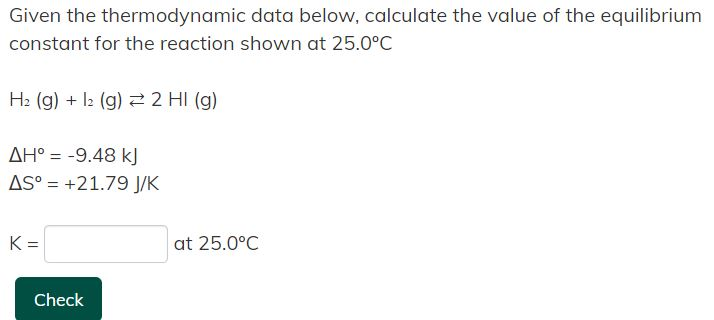Given the thermodynamic data below, calculate the value of the equilibrium constant for the reaction shown at 25.0°C H2(g) + 12 (g) = 2 HI(g) AH° = -9.48 kJ AS° = +21.79 J/K K= at 25.0°C Check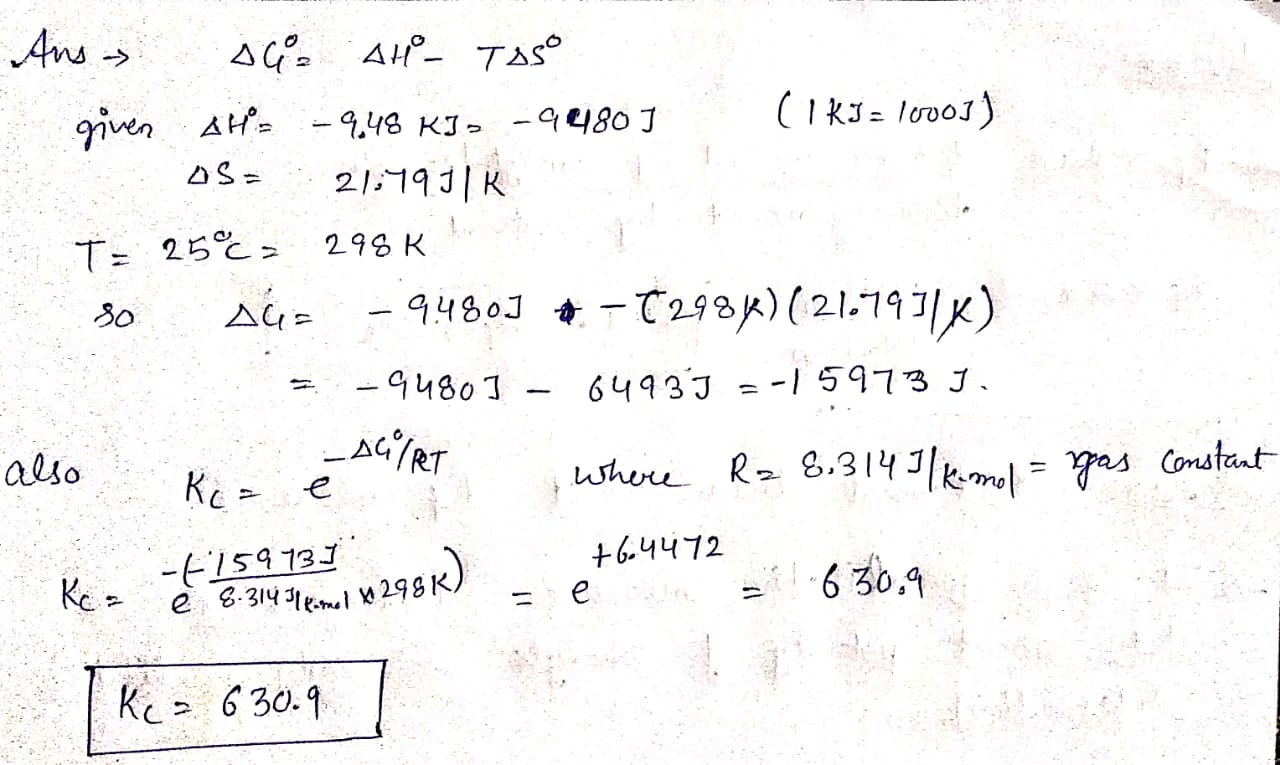Earn Coins

Coins can be redeemed for fabulous gifts.

Similar Homework Help Questions
• Given the thermodynamic data below, calculate the value of the equilibrium constant for the reaction shown...

Given the thermodynamic data below, calculate the value of the equilibrium constant for the reaction shown at 25.0ºC H₂ (g) + I₂ (g) ⇄ 2 HI (g) ΔHº = -9.48 kJ ΔSº = +21.79 J/K K = Answer at 25.0ºC

• Given the reference thermodynamic data below taken at 25°C, calculate the value of the equilibrium constant...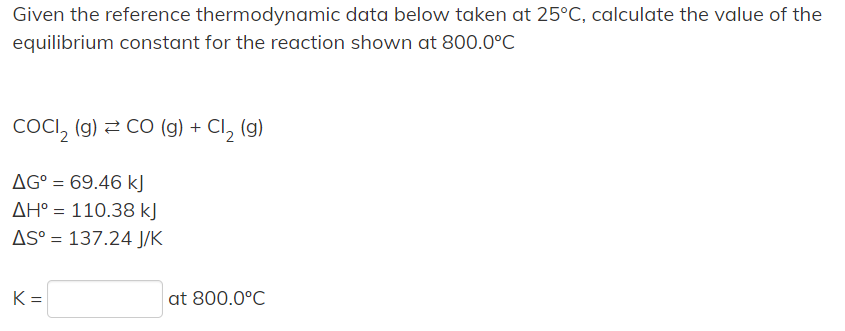Given the reference thermodynamic data below taken at 25°C, calculate the value of the equilibrium constant for the reaction shown at 800.0°C COCI, (g) 2 CO (g) + Cl2 (g) AG° = 69.46 kJ AH° = 110.38 kJ AS° = 137.24 J/K at 800.0°C

• problem #9 Calculate the equilibrium constant for the following reaction at 25°C. 2H2(g) + O2(g) 2H20cv)...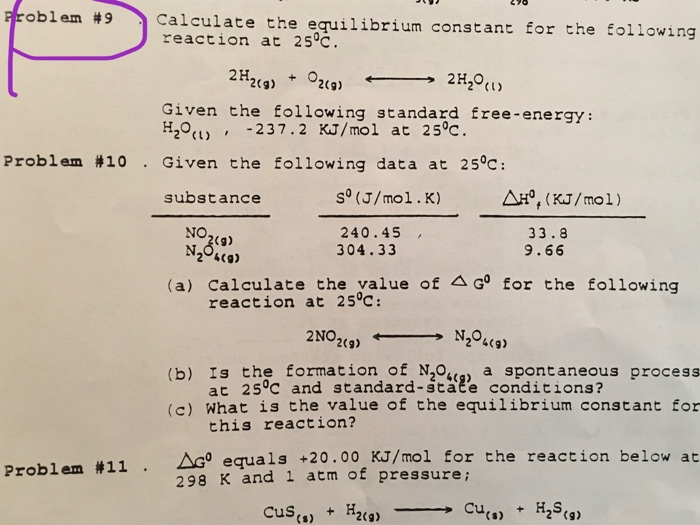problem #9 Calculate the equilibrium constant for the following reaction at 25°C. 2H2(g) + O2(g) 2H20cv) Given the following standard free-energy: H2O , -237.2 KJ/mol at 25°C. Problem #10. Given the following data at 25°C: substance sº (J/mol.K) AH', (KJ/mol) 240.45, 33.8 304.33 9.66 NO 249) N2O160) (a) Calculate the value of A Gº for the following reaction at 25°C: 2 NO2(9) N20 (9) (b) Is the formation of NO a spontaneous process at 25°C and standard-state conditions? (a) What...

• Use the data below, for 298.15 K, to calculate the thermodynamic equilibrium constant, kp, at 641...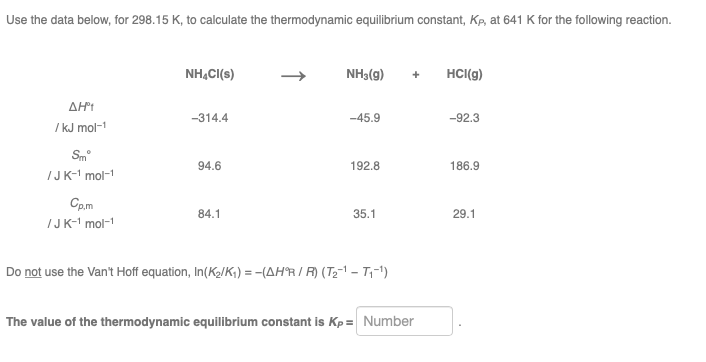Use the data below, for 298.15 K, to calculate the thermodynamic equilibrium constant, kp, at 641 K for the following reaction. NH4Cl(s) NH3(g) + HCl(g) ΔΗ /kJ mol-1 -314.4 -45.9 -92.3 Smº /JK-mol-1 94.6 192.8 186.9 Cp.m /JK-mol-1 84.1 35.1 29.1 Do not use the Van't Hoff equation, In(K/K) = -(AHR/R) (T2-1-T1-1) The value of the thermodynamic equilibrium constant is Kp = Number

• 1) Calculate the equilibrium constant at 138 K for the thermodynamic data in the previous question....

1) Calculate the equilibrium constant at 138 K for the thermodynamic data in the previous question. Notice that Keq is larger at the larger temperature for an endothermic reaction. 2) Endothermic reaction; increase in entropy Calculate the equilibrium constant at 40 K for a reaction with ΔHrxno = 10 kJ and ΔSrxno = 100 J/K.

• Use the data below, for 298.15 K, to calculate the thermodynamic equilibrium constant, kp, at 839...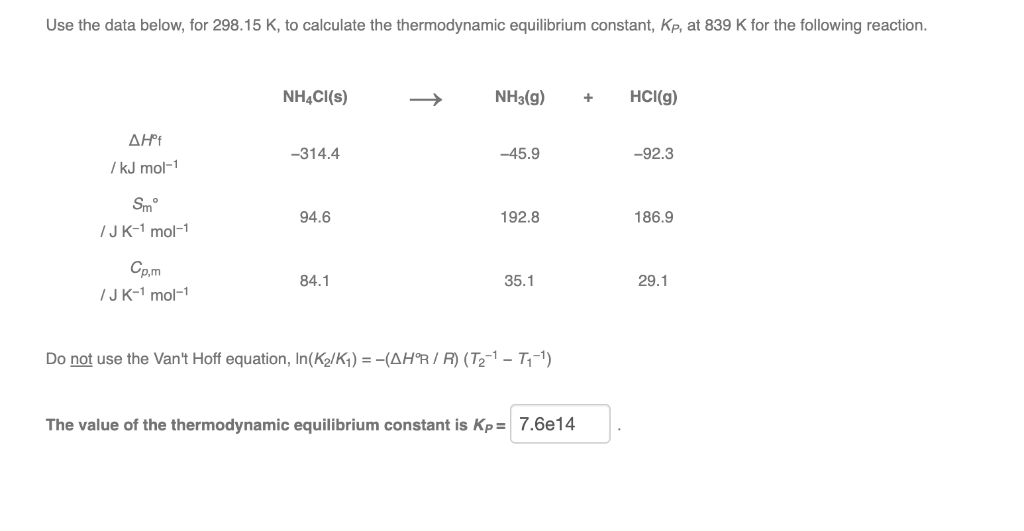Use the data below, for 298.15 K, to calculate the thermodynamic equilibrium constant, kp, at 839 K for the following reaction. NH4Cl(s) NH3(g) + HCl(g) ΔΗ 7 kJ mol-1 -314.4 -45.9 -92.3 Smº JK-1 mol-1 94.6 192.8 186.9 Cp,m 84.1 35.1 29.1 /JK-1 mol-1 Do not use the Van't Hoff equation, In(K /K1) = -(AHR/R) (T2-1 - 7,-1) The value of the thermodynamic equilibrium constant is Kp= 7.6e14

• Calculate the equilibrium constant from the standard free energy change. Using standard thermodynamic data (linked), calculate...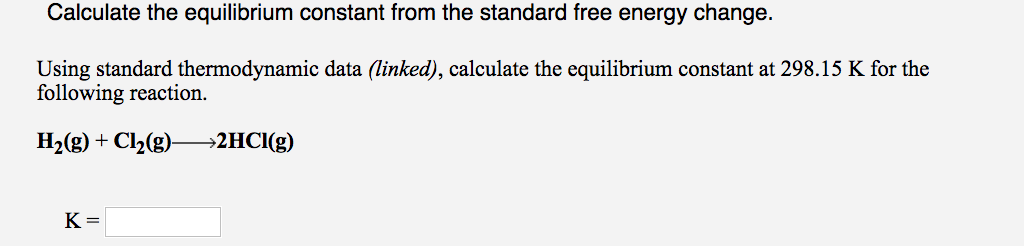Calculate the equilibrium constant from the standard free energy change. Using standard thermodynamic data (linked), calculate the equilibrium constant at 298.15 K for the following reaction. H2(g) + Cl2(g)—>2HCI(g) K=

• 4. The gas-phase dehydrogenation of propanol (A) to yield propionaldehyde (B) is in equilibrium at 230°C. Standard thermodynamic data for the reaction species at 298 K are: Propanol: Propionaldehyde...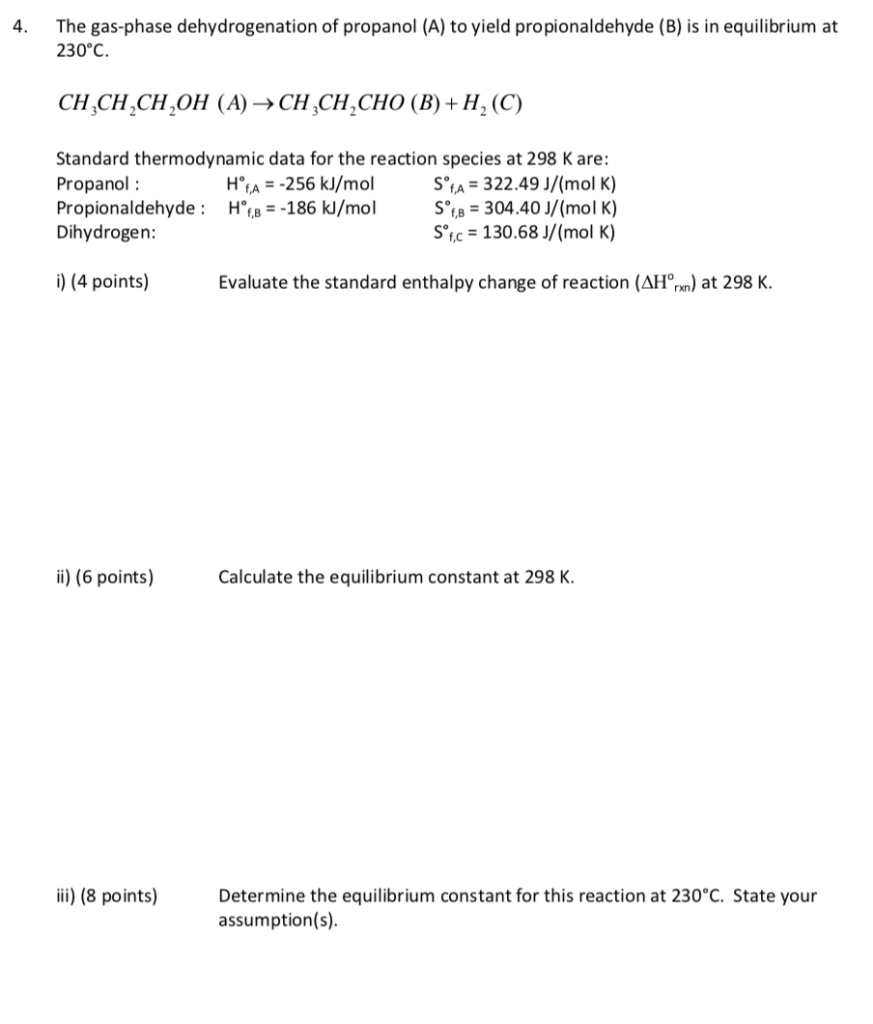4. The gas-phase dehydrogenation of propanol (A) to yield propionaldehyde (B) is in equilibrium at 230°C. Standard thermodynamic data for the reaction species at 298 K are: Propanol: Propionaldehyde : H.,--186 k/moi s'u-304.40 J/(mol K) Dihydrogen: H A -256 kJ/molSA 322.49 J/(mol K) Soc 130.68 J/(mol K) ) (4 points) Evaluate the standard enthalpy change of reaction (AH)at 298 K. ii (6 points Calculate the equilibrium constant at 298 K ) (8 points) Determine the equilibrium constant for this reaction...

• 5 through 20 PS. (Sec. 17.4) Using the following thermodynamic data at 25 °C, calculate change...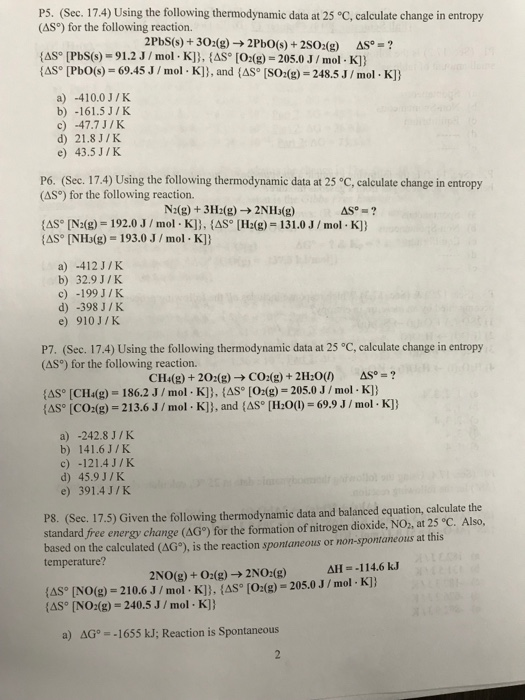5 through 20 PS. (Sec. 17.4) Using the following thermodynamic data at 25 °C, calculate change in entropy (ASo) for the following reaction. 2 PbS(s) +302(g) → 2Pb0(s) + 2SO2(g) Δ"-? [AS® [PbS(s)-91.2 J / mol . K] }, {AS。[O2(g) = 205.0 J / mol . K] } As*tPb0(s) = 69.45 J / mol . K] } , and {AS。[SO2(g)-248.5 J / miol-K] } a) -410.0J/K b) -161.5 J/K c) -47.7 J/K d) 21.8J/K e) 43.5 J/K P6. (Sec. 17.4)...

• Calculate the equilibrium constant from the standard free energy change. Using standard thermodynamic data (linked), calculate...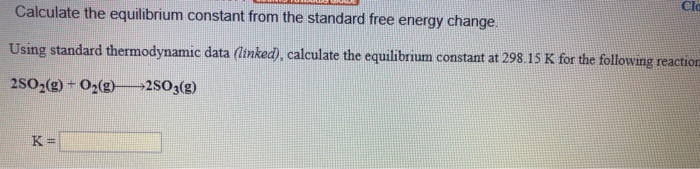Calculate the equilibrium constant from the standard free energy change. Using standard thermodynamic data (linked), calculate the equilibrium constant at 298.15 K for the following reaction 2502(g) + O2(0) 2503(9) AH°F (kJ/mol) AGⓇ, (kJ/mol sº (/mol K) 205.1 Oxygen 02(0) (9) O3(9) 249.2 231.7 161.1 142.7 163.2 238.9 AH°: (kJ/mol Agºr (kJ/mol) 5° (J/mol K) 31.8 0.3 0.3 278.8 3 3.0 167.8 33.0 - 15.0 Sulfur S(s, rhombic) S(s, monodinic) S(g) Sz"(aq) Sg(9) SzCl2(g) SF6(9) H2S(9) soz(9) SO3(0) 1 0....